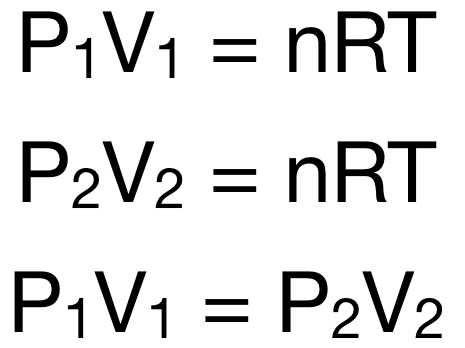Chemistry Practice Problems Chemistry Gas Laws Practice Problems Solution: A 12 Liter tank contains helium gas pressurized to...

🤓 Based on our data, we think this question is relevant for Professor Randles' class at UCF.

# Solution: A 12 Liter tank contains helium gas pressurized to 160 atm.How many 3-liter balloons could the 12 Liter helium tank fill?(Keep in mind that an "exhausted" helium tank is not empty. In other words, once the gas inside the tank reaches atmospheric pressure, it will no longer be able to fill balloons.)

###### Problem

A 12 Liter tank contains helium gas pressurized to 160 atm.

How many 3-liter balloons could the 12 Liter helium tank fill?

(Keep in mind that an "exhausted" helium tank is not empty. In other words, once the gas inside the tank reaches atmospheric pressure, it will no longer be able to fill balloons.)

###### Solution

Recall: The ideal gas law is given by the equation: PV = nRT

At constant temperature and moles of gas:The equation P1V1 = P2V2 is Boyle's Law, which states that the pressure of a certain amount of gas at a certain temperature is inversely proportional to its volume, i.e. the pressure decreases as volume increases.View Complete Written Solution

Chemistry Gas Laws

Chemistry Gas Laws

#### Q.  A sample of an ideal gas has a volume of 2.22 L at 279 K and 1.05 atm. Calculate the pressure when the volume is 1.73 L and the temperature is 303 K....

Solved • Sat May 12 2018 14:04:07 GMT-0400 (EDT)

Chemistry Gas Laws

#### Q. An ideal gas is allowed to expand from 7.60 L to 72.2 L at constant temperature. By what factor does the volume increase? The pressure will increase b...

Solved • Tue May 08 2018 09:05:34 GMT-0400 (EDT)

Chemistry Gas Laws

#### Q. What is the volume occupied by 18.6 g of argon gas at a pressure of 1.19 atm and a temperature of 394 K? Express your answer with the appropriate unit...

Solved • Tue Apr 24 2018 10:49:07 GMT-0400 (EDT)

Chemistry Gas Laws

#### Q. A 12 liter tank contains helium gas pressurized to 160 atm. What size tank would be needed to contain the same amount of helium at atmospheric pressur...

Solved • Fri Mar 30 2018 15:36:16 GMT-0400 (EDT)